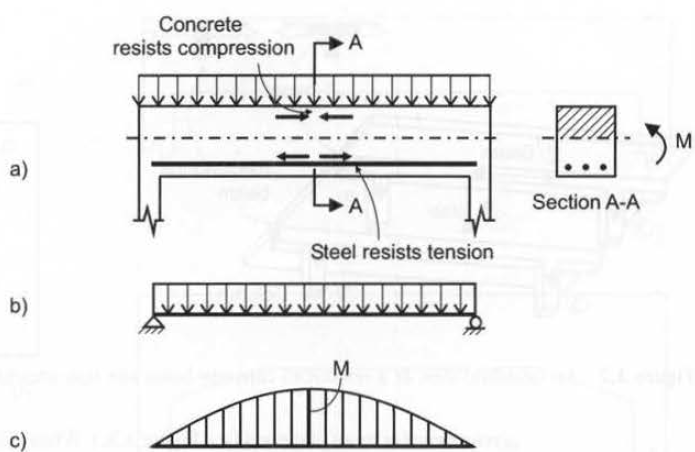# What is Flexure in Reinforced Concrete Members?

Flexure (bending) is associated with lateral deformation of a member under a transversely applied load. Consider a reinforced concrete beam subjected to the uniform load shown in Figure 1a. In cast-in-place concrete construction, beams act as a monolithic unit with the supporting columns. However, for design purposes the beam can be modeled as a simply supported member, as shown in Figure 1b. A bending moment diagram for this beam is shown in Figure 1c. The top portion of the beam is subjected to compression whereas the bottom is under tension. Concrete has a limited ability to carry tension and cracks once its tensile strength has been reached in the region of maximum bending moments (in this case at the beam midspan). To increase the bending resistance of a cracked beam, steel reinforcement (often called tension reinforcement or tension steel) is placed inside the beam near the bottom to resist the tensile stresses.Figure 1: Concept of flexure in reinforced concrete members: a) actual beam, showing the distribution of internal forces; b) beam model; c) bending moment diagram.

Did you know?

Positive bending causes tension at the bottom and compression at the top of a flexural member. The positive bending moment is shown on the bending moment diagram above the longitudinal axis of the member according to the North American convention.

Types of Flexural Members

An isometric view of a concrete floor structure subjected to gravity load is shown in Figure 2. The structure consists of a slab supported by beams, which are in turn supported by columns. In this case, beams are provided in one direction only. Consequently, the slab transfers the applied load in the direction perpendicular to the beams. This type of slab is called a one-way slab. One-way slabs are flexural members that behave essentially like wide beams. In cast-in-place concrete construction, beams are usually cast monolithically with the slab. The portion of the slab cast monolithically with a beam contributes to the beam moment resistance; this additional capacity is taken into consideration in the beam design. The term T-beam is used in this case because the slab and the beam form a T section for positive bending. In design practice, beams cast monolithically with the slab may be designed either as T-beams or as rectangular beams for positive bending. If the slab contribution is ignored, the beam is considered to act as a rectangular beam. The analysis of rectangular beams is simpler than that of T-beams. and it is a good starting point for understanding the flexural resistance of concrete members.Figure 2: An isometric view of reinforced concrete beam and slab structure
Reinforced concrete beams and slabs can be classified into simple and continuous structures. Continuous structures are statically indeterminate. Simple (or simply supported) structures span across two supports, as shown in Figure 3a, whereas continuous structures span across three (or more) supports (see Figure 3c). When subjected to a gravity load such as the uniform load in Figure 3a, only positive bending moments develop in simple structures (see Figure 3b), while both positive and negative bending moments develop in continuous structures subjected to the same load, as shown in Figure 3d. The deformed shape of the continuous beam subjected to a uniform load is shown in Figure 3e. The points where the curvature of the deflected shape changes from the sagging to the hogging shape are called inflection points (or points of contraflexure) and are denoted by IP on the diagram in Figure 3e. The same points correspond to the locations of zero moments on the bending moment diagram in Figure 3d.Figure 3: Simple vs continuous beams a) Simple beam; b) Bending moment diagram for a simple beam; c) Continuous beam; d) Bending moment diagram for a continuous beam; e) Deflection diagram for a continuous beam
In general, reinforced concrete members are characterized by regular cross-sectional dimensions. Members with constant cross-sectional dimensions within one span are called prismatic members (see Figure 4a). In some cases, the cross-sectional properties of a beam within a span are varied. Beams and slabs with variable cross-sectional dimensions along their length are called nonprismatic members. Haunched beams are nonprismatic members commonly found in design practice. These beams are characterized by larger cross-sectional dimensions in the support regions, which could also taper toward the midspan (see Figure 4b ). Haunched beams are sometimes considered to be a more effective design solution for longer-span continuous structures.Figure 4: Prismatic versus b) nonprismatic members: a) prismatic beam; b) nonprismatic (haunched) beam.

[blogger]

Engineeersdaily

Name

Email *

Message *